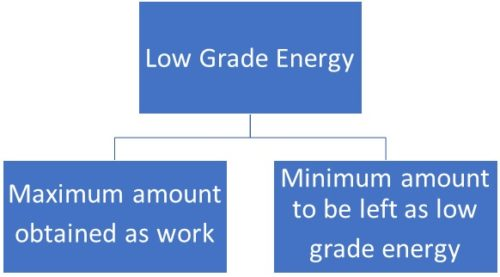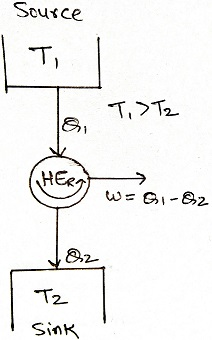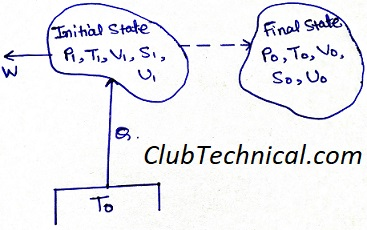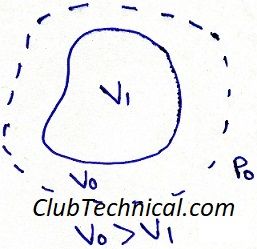# Exergy or Availability in Thermodynamics | Dead State in Thermodynamics

By | March 14, 2019
When a system is not in equilibrium (or dead state in this case) with its surroundings there is an opportunity in convert this departure from equilibrium to do some useful work.
For example;
1. If there is a difference between the temperature of system and surroundings then this temperature difference can be utilized to produce useful work with the help of a heat engine.
2. If a pressure difference is present between system and surrounding then this pressure difference can be utilized to get some useful work with the help of a turbine.

## Availability/ Available Energy/ Exergy

First of we all should know that availability, available energy and exergy are different names of the same thing.

The exergy of a given system is defined as the maximum useful work (total work minus P*dV work) that is obtained in a process in which the system comes to equilibrium with its surroundings. Exergy is a composite property which means that its value depends both on system and surroundings.

To understand what exergy is, we must first learn about the conversion principle of one form of energy into another.

Let’s take the example of heat and work. Both are forms of energy.

But we all know work can be totally converted into heat but heat can not be totally converted into work.

Which means

W → Q

But, Q → W + Q Rejected

Here comes the concept of high-grade and low-grade energy.

High grade energy is a type of energy which can be totally converted into work. For example, electrical energy, mechanical energy etc.

Low grade energy is the type of energy which can not be totally converted into work. For example, inter-molecular energy, thermal energy etc.The word availability is used in context of low grade energy.

The available energy (availability or exergy) of the low-grade energy, symbolizes the amount of work potential of that low-grade energy with respect to the given surroundings.

Which means availability is the maximum amount of useful work that can be extracted from a thermodynamic system with respect to the given surroundings.

And the minimum amount of energy which is left as low-grade energy in this energy conversion is called Anergy.

## Availability of conversion of thermal energy (Heat) into Work

Let’s consider a reversible heat engine.Maximum efficiency of a reversible heat engine, according to the Carnot’s theorem.

η = 1 – (T2/T1)

Hence, maximum work obtainable from a heat engine.

W Maximum = Q1 [1 – (T2/T1)]

From the above equation we can draw two conclusions.

1. Maximum work obtainable from a heat engine is directly proportional to the difference in its operating temperatures.
2. The amount of low grade energy (Anergy) which is to be rejected in conversion of low grade energy to high grade energy (work) is Q1(T2/T1)

## Dependence of exergy on source temperature

Let’s consider a heat engine which can take same quantity of heat from two different source temperatures (T1 and T2).

Where: T1 > T2 > T0Now, available energy (AE) obtained from the heat engine while obtaining heat from a source with temperature T1

AET1 = Q1 [1 – (T0/T1)]

Now, available energy (AE) obtained from the heat engine while obtaining heat from a source with temperature T2

AET2 = Q1 [1 – (T0/T2)]

From the above equation we can easily find that

AET1 > AET2

And also

AET1 – AET2 = Q1 * T0 [(1/T2) – (1-T1)]

Hence it is always advisable to extract heat from a high temperature source to obtain maximum work.

## Availability of a stationary closed thermodynamic system

Let’s consider a closed thermodynamic system (as shown in diagram).Initial states of the system are P1, V1, T1, S1, U1 and final states of the system are P0, V0, T0, S0, U0.

The system is taking heat from a source at temperature T0 and generating work W.

Where:

P: Pressure

T: Temperature

V: Volume

S: Entropy

U: Internal energy

Q = ΔU + W

Q = U0 – U1 + W   … (1)

From the principal of entropy change of a system we know that

(S0 – S1) – (Q/T0) ≥ 0

Q ≤ T0 (S0 – S1)   … (2)

From equation (1) and (2)

W ≤ U1 – U0 + T0 (S0 – S1)

W ≤ (U1 – T0S1) – (U0 – T0S0)   … (3)Above diagram shows a system with initial volume V1 and final volume V0 at pressure P0.

Useful work obtained from such a system would be

W Useful = W – P0 (V0 – V1)   … (4)

Where P0 (V0 – V1) is work done against the surroundings, which is not useful to us.

W Useful ≤ (U1 + P0V1 – T0S1) – (U0 + P0V0 – T0S0)

W Maximum = (U1 + P0V1 – T0S1) – (U0 + P0V0 – T0S0)

W Maximum = Ø1 – Ø0

Where:

U1 + P0V1 – T0S1 = Ø1

U0 + P0V0 – T0S0 = Ø0

Ø is known as availability function

## Dead state of a thermodynamic system

When a system comes in equilibrium to its surroundings, which means that the availability of the system is zero it is called in dead state. No useful work can be obtained from a system which is in dead state.

This site uses Akismet to reduce spam. Learn how your comment data is processed.